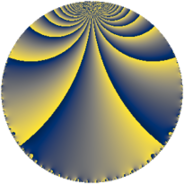# Properties

 Label 2736.3.o.jLevel $2736$ Weight $3$ Character orbit 2736.o Analytic conductor $74.551$ Analytic rank $0$ Dimension $4$ CM no Inner twists $4$

# Related objects

## Newspace parameters

 Level: $$N$$ $$=$$ $$2736 = 2^{4} \cdot 3^{2} \cdot 19$$ Weight: $$k$$ $$=$$ $$3$$ Character orbit: $$[\chi]$$ $$=$$ 2736.o (of order $$2$$, degree $$1$$, not minimal)

## Newform invariants

 Self dual: no Analytic conductor: $$74.5506003290$$ Analytic rank: $$0$$ Dimension: $$4$$ Coefficient field: $$\Q(\sqrt{-7}, \sqrt{10})$$ Defining polynomial: $$x^{4} - 2 x^{3} - 15 x^{2} + 16 x + 134$$ Coefficient ring: $$\Z[a_1, \ldots, a_{29}]$$ Coefficient ring index: $$2^{7}$$ Twist minimal: no (minimal twist has level 171) Sato-Tate group: $\mathrm{SU}(2)[C_{2}]$

## $q$-expansion

Coefficients of the $$q$$-expansion are expressed in terms of a basis $$1,\beta_1,\beta_2,\beta_3$$ for the coefficient ring described below. We also show the integral $$q$$-expansion of the trace form.

 $$f(q)$$ $$=$$ $$q -\beta_{1} q^{5} + 2 q^{7} +O(q^{10})$$ $$q -\beta_{1} q^{5} + 2 q^{7} + 2 \beta_{1} q^{11} + \beta_{3} q^{13} + ( 9 + \beta_{3} ) q^{19} -\beta_{1} q^{23} + 15 q^{25} -\beta_{2} q^{29} + \beta_{3} q^{31} -2 \beta_{1} q^{35} -3 \beta_{3} q^{37} + \beta_{2} q^{41} -34 q^{43} + 13 \beta_{1} q^{47} -45 q^{49} -3 \beta_{2} q^{53} -80 q^{55} + \beta_{2} q^{59} + 86 q^{61} -5 \beta_{2} q^{65} + 4 \beta_{3} q^{67} -6 \beta_{2} q^{71} -102 q^{73} + 4 \beta_{1} q^{77} + 7 \beta_{3} q^{79} -4 \beta_{1} q^{83} + 6 \beta_{2} q^{89} + 2 \beta_{3} q^{91} + ( -9 \beta_{1} - 5 \beta_{2} ) q^{95} -2 \beta_{3} q^{97} +O(q^{100})$$ $$\operatorname{Tr}(f)(q)$$ $$=$$ $$4q + 8q^{7} + O(q^{10})$$ $$4q + 8q^{7} + 36q^{19} + 60q^{25} - 136q^{43} - 180q^{49} - 320q^{55} + 344q^{61} - 408q^{73} + O(q^{100})$$

Basis of coefficient ring in terms of a root $$\nu$$ of $$x^{4} - 2 x^{3} - 15 x^{2} + 16 x + 134$$:

 $$\beta_{0}$$ $$=$$ $$1$$ $$\beta_{1}$$ $$=$$ $$($$$$-4 \nu^{3} + 6 \nu^{2} + 110 \nu - 56$$$$)/47$$ $$\beta_{2}$$ $$=$$ $$($$$$32 \nu^{3} - 48 \nu^{2} - 128 \nu + 72$$$$)/47$$ $$\beta_{3}$$ $$=$$ $$2 \nu^{2} - 2 \nu - 16$$
 $$1$$ $$=$$ $$\beta_0$$ $$\nu$$ $$=$$ $$($$$$\beta_{2} + 8 \beta_{1} + 8$$$$)/16$$ $$\nu^{2}$$ $$=$$ $$($$$$8 \beta_{3} + \beta_{2} + 8 \beta_{1} + 136$$$$)/16$$ $$\nu^{3}$$ $$=$$ $$($$$$12 \beta_{3} + 29 \beta_{2} + 44 \beta_{1} + 200$$$$)/16$$

## Character values

We give the values of $$\chi$$ on generators for $$\left(\mathbb{Z}/2736\mathbb{Z}\right)^\times$$.

 $$n$$ $$1009$$ $$1217$$ $$1711$$ $$2053$$ $$\chi(n)$$ $$-1$$ $$1$$ $$1$$ $$1$$

## Embeddings

For each embedding $$\iota_m$$ of the coefficient field, the values $$\iota_m(a_n)$$ are shown below.

For more information on an embedded modular form you can click on its label.

Label $$\iota_m(\nu)$$ $$a_{2}$$ $$a_{3}$$ $$a_{4}$$ $$a_{5}$$ $$a_{6}$$ $$a_{7}$$ $$a_{8}$$ $$a_{9}$$ $$a_{10}$$
721.1
 3.66228 − 1.32288i 3.66228 + 1.32288i −2.66228 + 1.32288i −2.66228 − 1.32288i
0 0 0 −6.32456 0 2.00000 0 0 0
721.2 0 0 0 −6.32456 0 2.00000 0 0 0
721.3 0 0 0 6.32456 0 2.00000 0 0 0
721.4 0 0 0 6.32456 0 2.00000 0 0 0
 $$n$$: e.g. 2-40 or 990-1000 Significant digits: Format: Complex embeddings Normalized embeddings Satake parameters Satake angles

## Inner twists

Char Parity Ord Mult Type
1.a even 1 1 trivial
3.b odd 2 1 inner
19.b odd 2 1 inner
57.d even 2 1 inner

## Twists

By twisting character orbit
Char Parity Ord Mult Type Twist Min Dim
1.a even 1 1 trivial 2736.3.o.j 4
3.b odd 2 1 inner 2736.3.o.j 4
4.b odd 2 1 171.3.c.e 4
12.b even 2 1 171.3.c.e 4
19.b odd 2 1 inner 2736.3.o.j 4
57.d even 2 1 inner 2736.3.o.j 4
76.d even 2 1 171.3.c.e 4
228.b odd 2 1 171.3.c.e 4

By twisted newform orbit
Twist Min Dim Char Parity Ord Mult Type
171.3.c.e 4 4.b odd 2 1
171.3.c.e 4 12.b even 2 1
171.3.c.e 4 76.d even 2 1
171.3.c.e 4 228.b odd 2 1
2736.3.o.j 4 1.a even 1 1 trivial
2736.3.o.j 4 3.b odd 2 1 inner
2736.3.o.j 4 19.b odd 2 1 inner
2736.3.o.j 4 57.d even 2 1 inner

## Hecke kernels

This newform subspace can be constructed as the intersection of the kernels of the following linear operators acting on $$S_{3}^{\mathrm{new}}(2736, [\chi])$$:

 $$T_{5}^{2} - 40$$ $$T_{7} - 2$$

## Hecke characteristic polynomials

$p$ $F_p(T)$
$2$ $$T^{4}$$
$3$ $$T^{4}$$
$5$ $$( -40 + T^{2} )^{2}$$
$7$ $$( -2 + T )^{4}$$
$11$ $$( -160 + T^{2} )^{2}$$
$13$ $$( 280 + T^{2} )^{2}$$
$17$ $$T^{4}$$
$19$ $$( 361 - 18 T + T^{2} )^{2}$$
$23$ $$( -40 + T^{2} )^{2}$$
$29$ $$( 448 + T^{2} )^{2}$$
$31$ $$( 280 + T^{2} )^{2}$$
$37$ $$( 2520 + T^{2} )^{2}$$
$41$ $$( 448 + T^{2} )^{2}$$
$43$ $$( 34 + T )^{4}$$
$47$ $$( -6760 + T^{2} )^{2}$$
$53$ $$( 4032 + T^{2} )^{2}$$
$59$ $$( 448 + T^{2} )^{2}$$
$61$ $$( -86 + T )^{4}$$
$67$ $$( 4480 + T^{2} )^{2}$$
$71$ $$( 16128 + T^{2} )^{2}$$
$73$ $$( 102 + T )^{4}$$
$79$ $$( 13720 + T^{2} )^{2}$$
$83$ $$( -640 + T^{2} )^{2}$$
$89$ $$( 16128 + T^{2} )^{2}$$
$97$ $$( 1120 + T^{2} )^{2}$$